# Pencils

Mrs. P is ordering pencils for next year's class. Mrs. P expects to have twenty-four students in the class. Each student will use twelve pencils during the year. Pencils come in boxes of one hundred forty-four pencils. How many pencils will Mrs. P need for the next year's class? How many boxes of pencils should Mrs. P order to have enough for next year's class? Show all your mathematical thinking.

Assessment

## Plan

#### Formal Mathematical Language and Symbolic Notation

A student may independently select a printed number line, number chart, ten frames, graph paper, etc. as they work on a task.

### Suggested materials

Engagement Image:

Teachers may project the image below to launch this task for their students, define nouns, promote discussion, access prior knowledge, and inspire engagement and problem solving.

## Solutions tabs

### Expert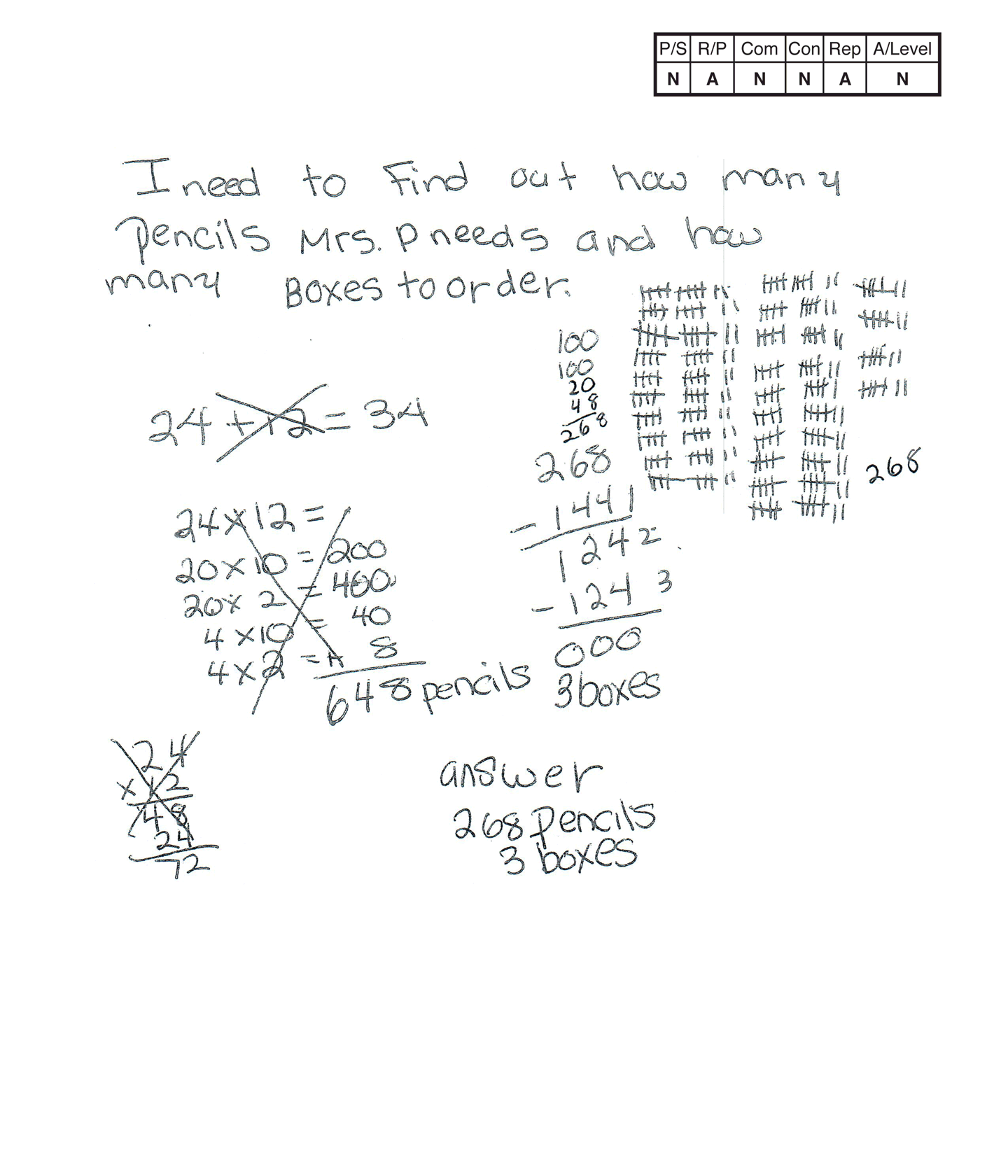This student does not meet the standard.

### Scoring Rationale

#### Novice

The student’s strategy to use tally marks in 20 sets of 12 and four sets of seven would not work to solve the first part of the task. The student’s answer, “268 pencils,” is not correct. The student’s strategy of subtracting 144 pencils from 268 pencils and counting the subtrahend as one box for a total of three boxes would not work to solve the second part of the task.

#### Apprentice

The student does not demonstrate understanding of the major underlying concept of the task. The student is not able to multiply two two-digit numbers using strategies based on place value and the properties of operations. The student does show partial understanding. It appears that the student is using 12 tally marks to represent 12 pencils for each of 20 students. But the student uses seven tally marks for each of the remaining four students.

#### Novice

The student does not use any mathematical language in their solution.

#### Novice

The student does not make a mathematically relevant connection.

#### Apprentice

It appears that the student is attempting a representation by making ten groups of twelve and another 10 groups of 12 to indicate 12 pencils per 20 students. The student uses seven tally marks and not 12 for the remaining four students’ pencils. The student does not provide a key, labels or text to define the tally marks.

#### Novice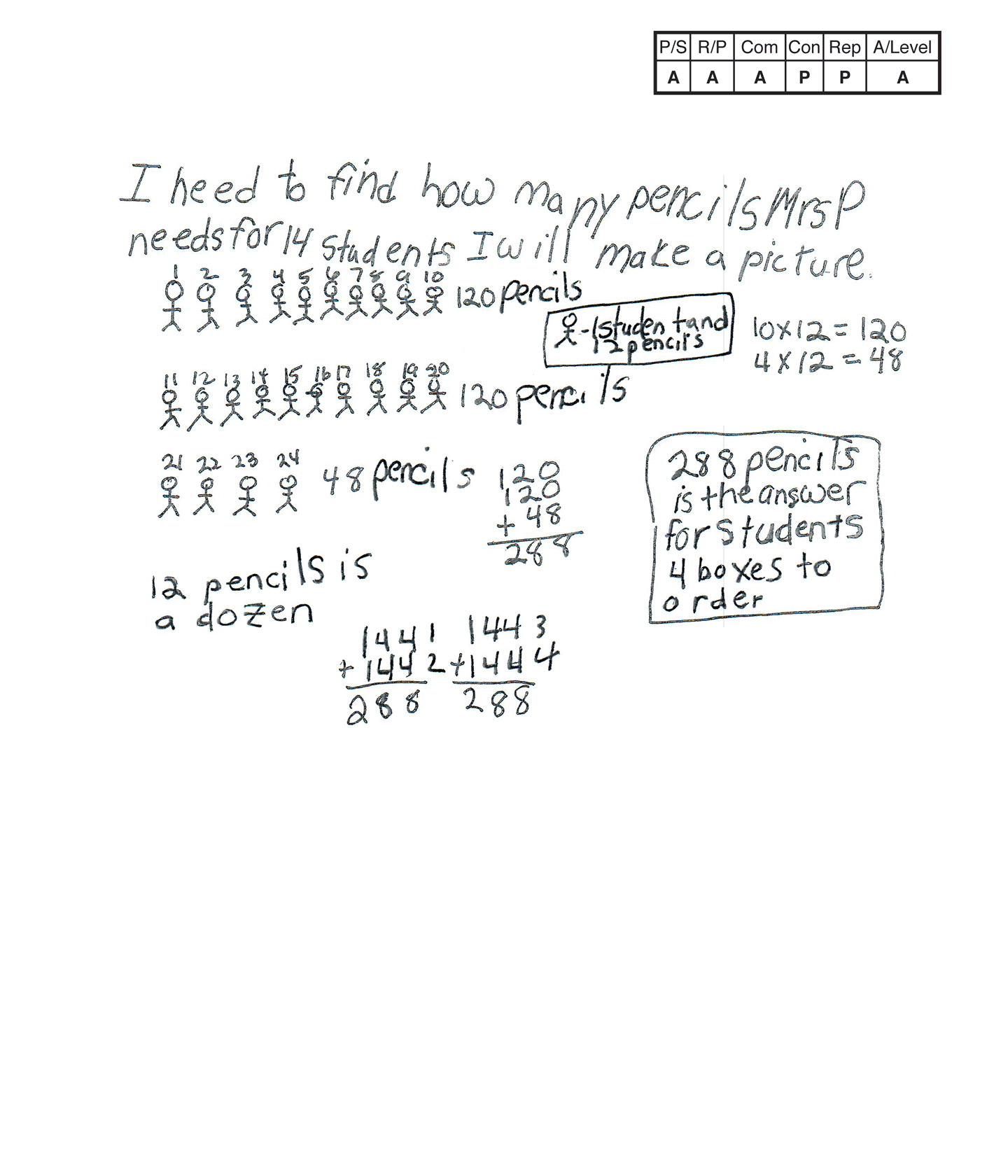This student does not meet the standard.

### Scoring Rationale

#### Apprentice

The student’s strategy of using a diagram to show the 288 pencils needed for 24 students works to solve the first part of the task. The student’s answer, “288 pencils is the answer for students,” is correct. The student’s strategy of adding 144 twice and counting the four 144s as four boxes would not work to solve the second part of the task. The student’s answer, “4 boxes to order,” is not correct.

#### Apprentice

The student demonstrates understanding of the underlying concepts of the task. The student is able to multiply two two-digit numbers, using strategies based on place value and the properties of operations. The student does not show correct understanding of applying addition or subtraction to determine how many boxes of pencils are needed to supply 288 pencils to 24 students.

#### Apprentice

The student correctly uses the mathematical term dozen.

#### Practitioner

The student makes the mathematically relevant observation, “12 pencils is a dozen.”

#### Practitioner

The student’s diagram is appropriate and accurate. The student defines the figure as “1 student and 12 pencils.” The student correctly diagrams 24 students for a total of 288 pencils.

#### Apprentice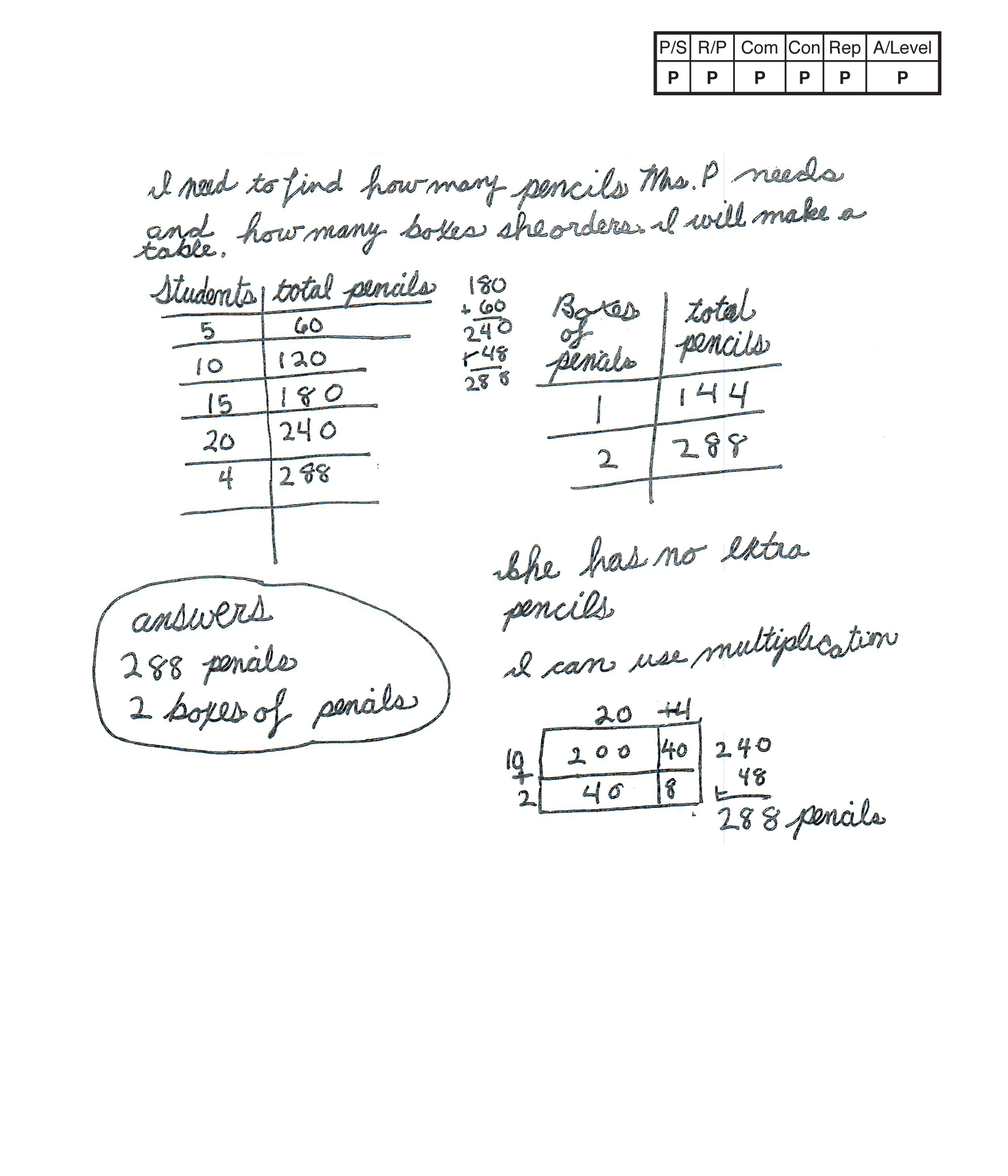This student meets the standard.

### Scoring Rationale

#### Practitioner

The student’s strategy of using tables to indicate the total number of pencils and boxes of pencils Mrs. P needs to order works to solve the task. The student’s answer, “288 pencils, 2 boxes of pencils,” is correct.

#### Practitioner

The student demonstrates understanding of the underlying concepts of the task. The student is able to multiply two two-digit numbers, using strategies based on place value and the properties of operations.

#### Practitioner

The student correctly uses the mathematical terms table, total, multiplication.

#### Practitioner

The student makes the mathematically relevant observation, “She has no extra pencils.” The student uses the area model for multiplication to find 288 pencils.

#### Practitioner

The student’s diagram tables are appropriate to the task and accurate. The student provides correct labels and all entered data is correct. The student’s area model is appropriate to the task and accurate.

#### Practitioner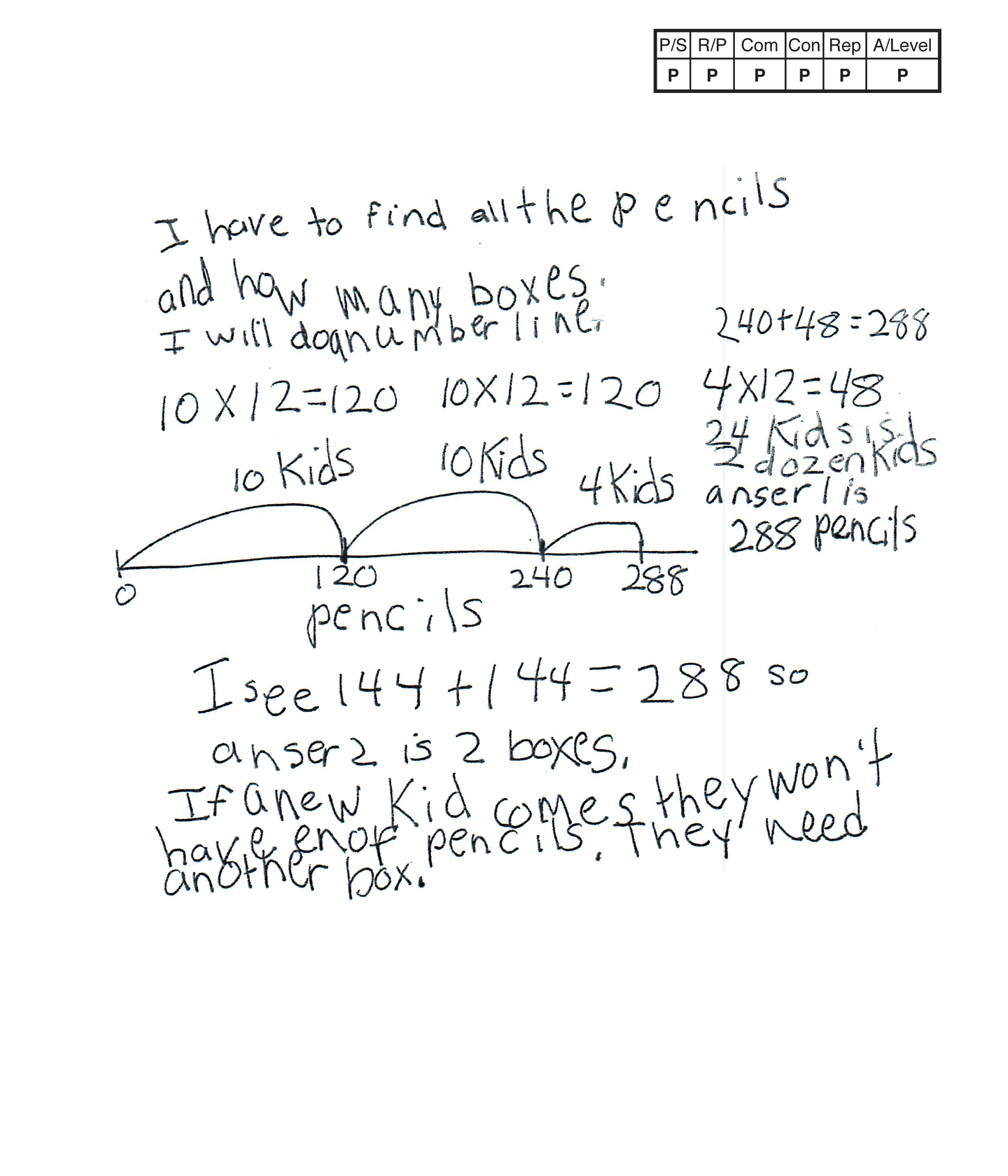This student meets the standard.

### Scoring Rationale

#### Practitioner

The student’s strategy of using a number line to indicate the total number of pencils Mrs. P needs to order works to solve the task. The student’s answer, “anser 1 is 288 pencils,” is correct. The student's use of addition works to solve the second part of the task. The student’s answer, “anser 2 is 2 boxes,” is correct.

#### Practitioner

The student demonstrates understanding of the underlying concepts of the task. The student is able to multiply two two-digit numbers, using strategies based on place value and the properties of operations.

#### Practitioner

The student correctly uses the mathematical terms number line, dozen.

#### Practitioner

The student makes the mathematically relevant observation, “If a new kid comes they won’t have enof pencils. They need another box.”

#### Practitioner

The student’s number line is appropriate to the task and accurate. The student labels the numbers as representing pencils and the jumps as two groups of 10 kids and one group of four kids.

#### Practitioner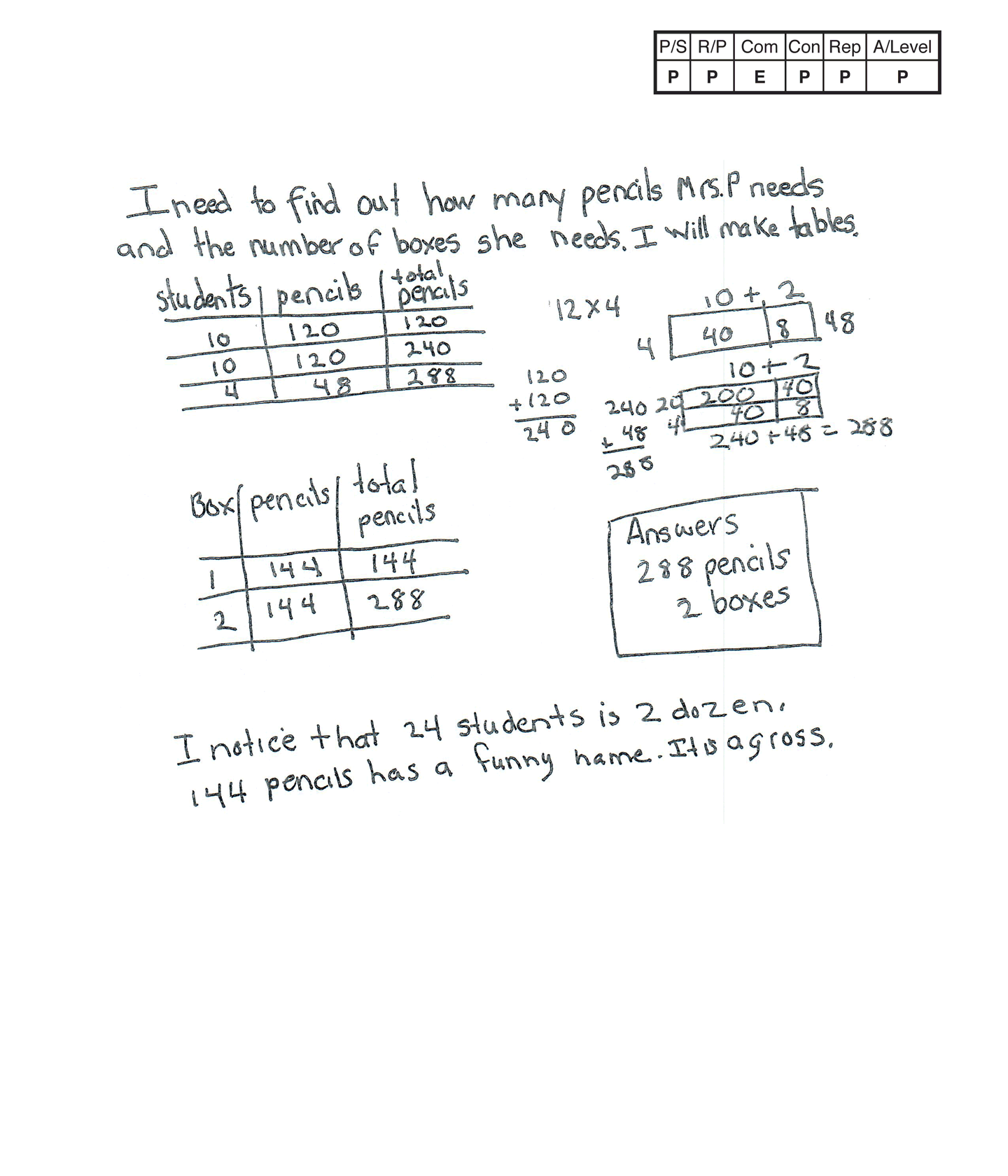This student meets the standard.

### Scoring Rationale

#### Practitioner

The student’s strategy of using tables to indicate the total number of pencils and boxes Mrs. P needs to order works to solve the task. The student’s answer, “288 pencils, 2 boxes” is correct.

#### Practitioner

The student demonstrates understanding of the underlying concepts of the task. The student is able to multiply two two-digit numbers, using strategies based on place value and the properties of operations.

#### Expert

The student correctly uses the mathematical terms tables, total, dozen, gross. Gross is considered an Expert math term as it is not common to grade four instruction.

#### Practitioner

The student makes the mathematically relevant observation, “I notice that 24 students is 2 dozen,” and, “144 pencils has a funny name. It is a gross.”

#### Practitioner

The student’s tables are appropriate to the task and accurate. The student labels each column correctly and the entered data is accurate. The student’s area models are appropriate to the task and accurate.

#### Practitioner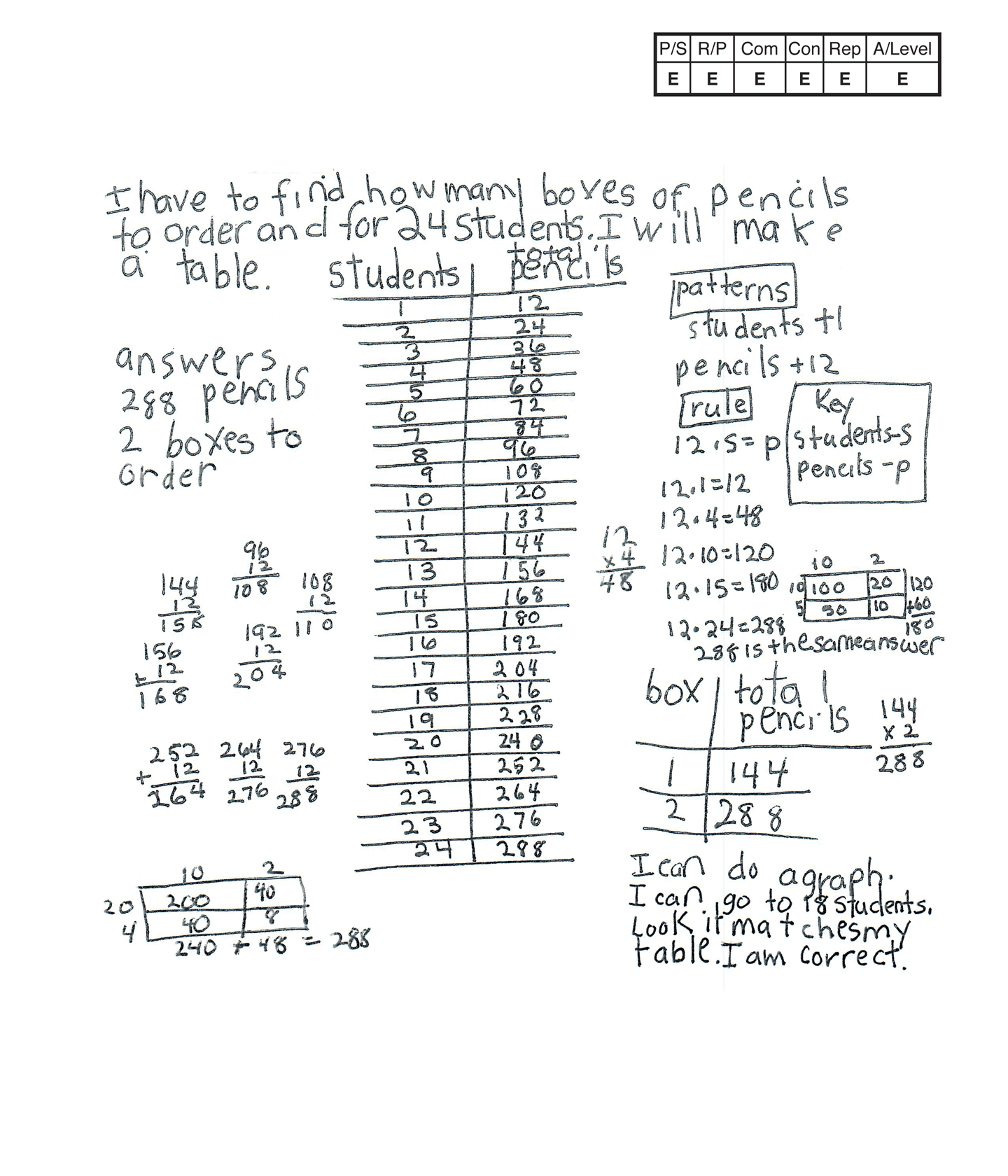,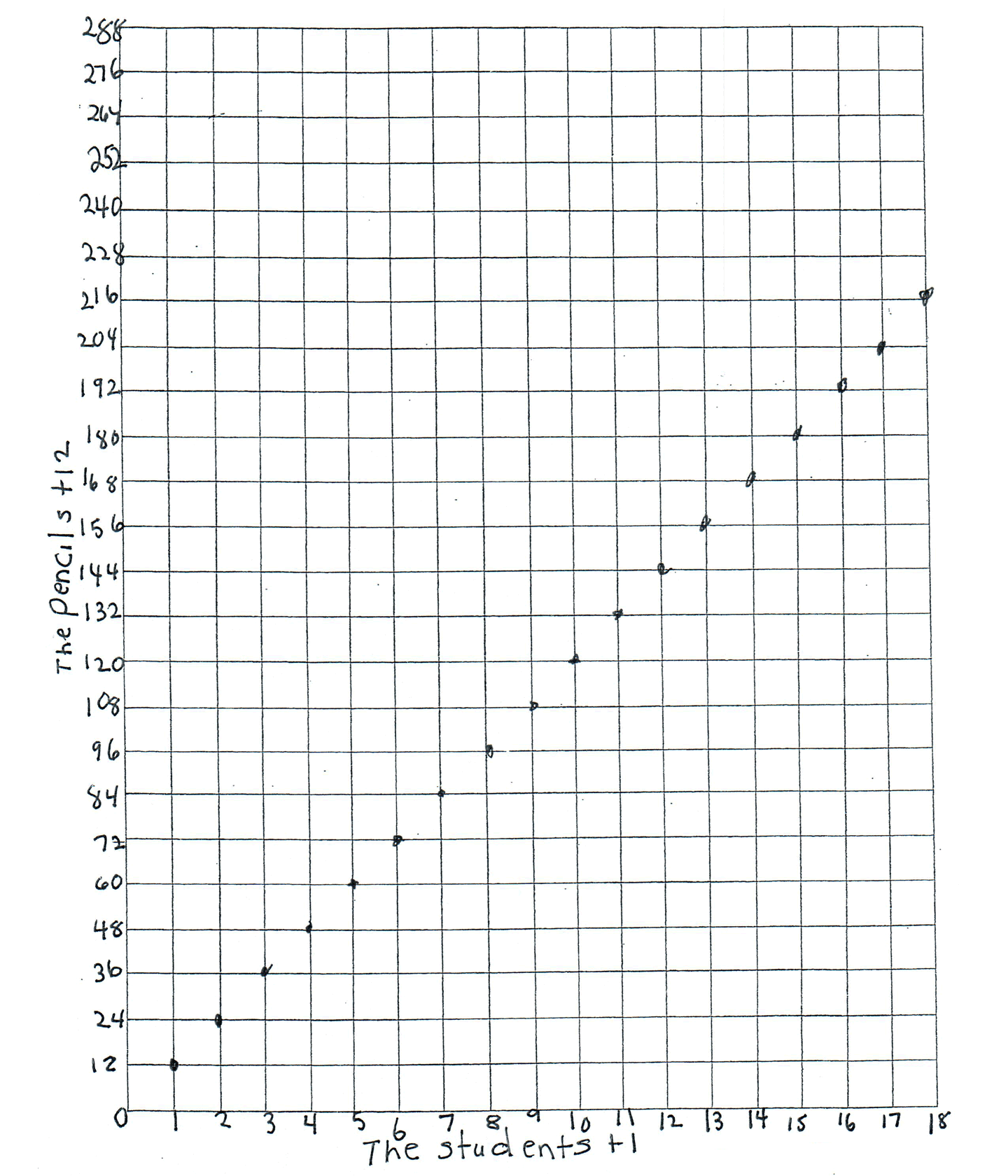This student exceeds the standard.

### Scoring Rationale

#### Expert

The student’s strategy of using tables to indicate the total number of pencils and boxes Mrs. P needs to order works to solve the task. The student’s answer, “288 pencils, 2 boxes to order,” is correct. The student generalizes and applies a rule for finding the number of pencils for any number of students.

#### Expert

The student demonstrates understanding of the underlying concepts of the task. The student is able to multiply two two-digit numbers, using strategies based on place value and the properties of operations. The student uses a graph and a rule to justify that their answer is correct.

#### Expert

The student correctly uses the mathematical terms table, dozen, patterns, rule, key, total, graph. The student correctly uses the symbolic mathematical notation 12 · s = p with the variables defined in a key.

#### Expert

The student makes the mathematically relevant Practitioner observations, “12 is a dozen,” and, “patterns, students + 1, pencils +2.” The student makes Expert connections. The student generalizes a rule, 12 · s = p and applies the rule for one, four, 10, 15, and 100 students. The student uses the rule to justify that their answer for total pencils needed to be ordered is 288. The student states, “288 is the same answer.” The student creates a graph to show the patterns in their table and to again find 288 pencils for 24 students. The student states, “I can do a graph. I can go to 18 students. Look it matches my table. I am correct.”

#### Expert

The student’s table is appropriate to the task and accurate. The student labels each column correctly and the entered data is accurate. The student refers to their table to generalize and apply a rule to verify that the student’s area models are appropriate to the task and accurate.